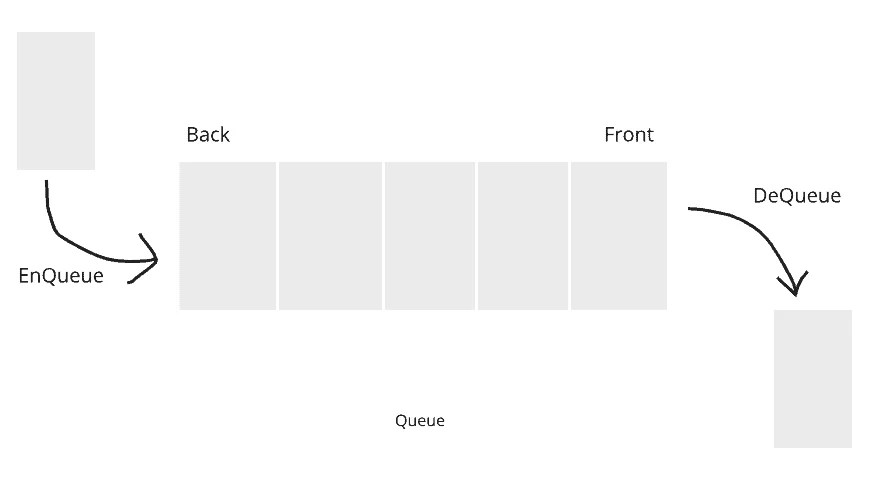# Queue Data Structure in Java

0
614Same as Stack, Queue is a linear Data Structure. But it stores the elements in FIFO(First In First Out) manner. In this post, we will learn about the Queue implementation in Java.

As we know, Java had an already built-in Interface called Collection. In the Collection Interface, the Queue is already implemented for our ease. In this post, we will learn a way how Developers built their own Queue implementation in Java to deep dive into the Queue concepts.

You will learn about the Queue Interface and their built-in methods in the later posts. If you want to learn Queue Collection Framework now you should follow the link.

Now, In Queue we are talking about the examples. Example of Queue is anything, It always in a manner where element comes in first and leave/removed first.

Unlike Stack, In Queue we will insert elements from one end and delete the elements from another end.

## Example of Queue:

A good real-time example of Queue is a line of students who are in a line to take the admission in college. The student comes first for admission in the line gets the admission first and leaves the line first.

Some other example is Sending request to the server.

## Applications of Queue:

• CPU Scheduling: Various CPU scheduling algorithms make use of this data structure to implement multiprocessing.
• Synchronization during data transfer: Asynchronous data transfers use a queue to keep track of the data. This includes pipes and IO buffers.

## Overflow/Underflow Conditions:

We will always check Overflow and Underflow conditions at the time of insertion/Enqueue or deletion/Dequeue of the element from the Queue.

A Queue may be limited with the size (in Array) depending on the implementation so we need to check the Queue, not in any of the condition i.e; Overflow and Underflow so we can perform the operations on it.

### Overflow Condition:

It is always checked at the time of Enqueue of the element in the Queue. If the elements we inserted into the queue exceed the defined size then Queue Overflow message shows.

```if (rear == SIZE-1){
//Overflow Message
System.out.print("Queue Overflow. No Space to enqueue the element.");
}```

### Underflow Condition:

Underflow condition always checks while we are Dequeue the element from the Queue. we check if there is an element in the Queue or not if there is an element then we simply remove the element otherwise we show Underflow message.

```if (front == rear){
//Underflow Message
System.out.print("Queue Underflow. No element for Dequeue.");
}```

## Queue Operations in Java:

There are 2 operations we can perform in the Queue:

### Enqueue:

Enqueue method is used to add a new element to the Queue. The element is always added at the end/back/rear of the Queue. (see in the above picture).

Enqueue Operation always checked by Overflow Condition first. If the Queue is not over by its size then we will insert the new element.

### Dequeue:

Dequeue removes an element from the Queue. the element is always removed from the front of the Queue (see in the above picture).

### Front and Rear Pointer:

To efficiently add or remove data from the queue, two special pointers are used which keep track of the first and last element.

A front pointer is always pointing the element which is dequeue from the Queue.

Rear/Back/End pointer always points to the position where the element enqueue.

## Time Complexities for Queue Operations:

In Queue, there are 4 operations we can mainly perform:

### Access :

``O(n)``

### Search:

``O(n)``

### Insertion:

``O(1)``

### Deletion:

``O(1)``

## Types of Queue:

• Double-Ended queue (Deque)
• Circular Queue (Circular Buffer)
• Priority Queue

### Double-Ended Queue:

In a standard queue, we can only enqueue element done from the back and deletion only from the front. A double-ended queue allows for Enqueue and Dequeue from both ends.

### Circular Queue:

A circular queue uses a single, fixed-size buffer as if it were connected end-to-end like a circle.

In case of a circular queue, `front` the pointer will always point to the front of the queue, and rear the pointer will always point to the end of the queue.

### Priority Queue:

A priority queue assigns a priority to each element in the queue. This priority determines which element, we will delete and processed first.

• An element with the highest priority gets processed first.
• If there exist two elements with the same priority, then the order of which the element was inserted is considered.

#### Hope you like this post.

If you have any doubt please feel free to comment below.

You may also learn these to build the Knowledge: